# 532 and Level 3

532 is the sum of consecutive prime numbers 263 and 269.

532 is also the 19th pentagonal number.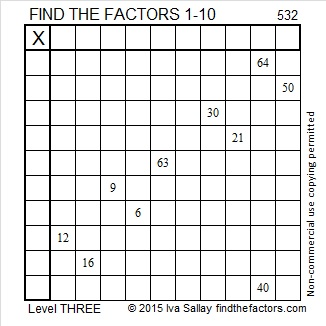Print the puzzles or type the solution on this excel file: 10 Factors 2015-06-22

—————————————————————————————————

• 532 is a composite number.
• Prime factorization: 532 = 2 x 2 x 7 x 19, which can be written 532 = (2^2) x 7 x 19
• The exponents in the prime factorization are 1, 2, and 1. Adding one to each and multiplying we get (2 + 1)(1 + 1)(1 + 1) = 3 x 2 x 2 = 12. Therefore 532 has exactly 12 factors.
• Factors of 532: 1, 2, 4, 7, 14, 19, 28, 38, 76, 133, 266, 532
• Factor pairs: 532 = 1 x 532, 2 x 266, 4 x 133, 7 x 76, 14 x 38, or 19 x 28
• Taking the factor pair with the largest square number factor, we get √532 = (√4)(√133) = 2√133 ≈ 23.065125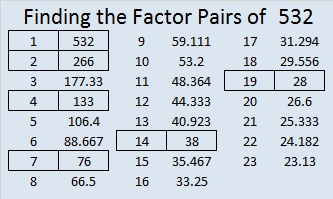—————————————————————————————————

A Logical Approach to solve a FIND THE FACTORS puzzle: Find the column or row with two clues and find their common factor. Write the corresponding factors in the factor column (1st column) and factor row (top row).  Because this is a level three puzzle, you have now written a factor at the top of the factor column. Continue to work from the top of the factor column to the bottom, finding factors and filling in the factor column and the factor row one cell at a time as you go.# 499 and Level 5

499 is the sum of a nice bunch of consecutive prime numbers:

• 3 + 5 + 7 + 11 + 13 + 17 + 19 + 23 + 29 + 31 + 37 + 41 + 43 + 47 + 53 + 59 + 61 = 499. That’s 17 consecutive primes.Print the puzzles or type the solution on this excel file: 12 Factors 2015-05-18

—————————————————————————————————

• 499 is a prime number.
• Prime factorization: 499 is prime.
• The exponent of prime number 499 is 1. Adding 1 to that exponent we get (1 + 1) = 2. Therefore 499 has exactly 2 factors.
• Factors of 499: 1, 499
• Factor pairs: 499 = 1 x 499
• 499 has no square factors that allow its square root to be simplified. √499 ≈ 22.3383079How do we know that 499 is a prime number? If 499 were not a prime number, then it would be divisible by at least one prime number less than or equal to √499 ≈ 22.3383. Since 499 cannot be divided evenly by 2, 3, 5, 7, 11, 13, 17, or 19, we know that 499 is a prime number.

—————————————————————————————————# 497 and Level 3

497 is the sum of a five consecutive prime numbers: 89 + 97 + 101 + 103 + 107 = 497.Print the puzzles or type the solution on this excel file: 12 Factors 2015-05-18

—————————————————————————————————

• 497 is a composite number.
• Prime factorization: 497 = 7 x 71
• The exponents in the prime factorization are 1 and 1. Adding one to each and multiplying we get (1 + 1)(1 + 1) = 2 x 2 = 4. Therefore 497 has exactly 4 factors.
• Factors of 497: 1, 7, 71, 497
• Factor pairs: 497 = 1 x 497 or 7 x 71
• 497 has no square factors that allow its square root to be simplified. √497 ≈ 22.2934968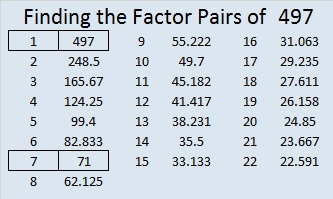—————————————————————————————————

A Logical Approach to solve a FIND THE FACTORS puzzle: Find the column or row with two clues and find their common factor. Write the corresponding factors in the factor column (1st column) and factor row (top row).  Because this is a level three puzzle, you have now written a factor at the top of the factor column. Continue to work from the top of the factor column to the bottom, finding factors and filling in the factor column and the factor row one cell at a time as you go.# 479 and Level 6

479 is the sum of nine consecutive prime numbers.

37 + 41 + 43 + 47 + 53 + 59 + 61 + 67 + 71 = 479.Print the puzzles or type the solution on this excel file: 10 Factors 2015-04-27

—————————————————————————————————

• 479 is a prime number.
• Prime factorization: 479 is prime.
• The exponent of prime number 479 is 1. Adding 1 to that exponent we get (1 + 1) = 2. Therefore 479 has exactly 2 factors.
• Factors of 479: 1, 479
• Factor pairs: 479 = 1 x 479
• 479 has no square factors that allow its square root to be simplified. √479 ≈ 21.88607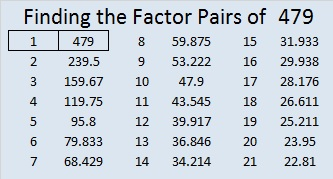How do we know that 479 is a prime number? If 479 were not a prime number, then it would be divisible by at least one prime number less than or equal to √479 ≈ 21.8861. Since 479 cannot be divided evenly by 2, 3, 5, 7, 11, 13, 17, or 19, we know that 479 is a prime number.

—————————————————————————————————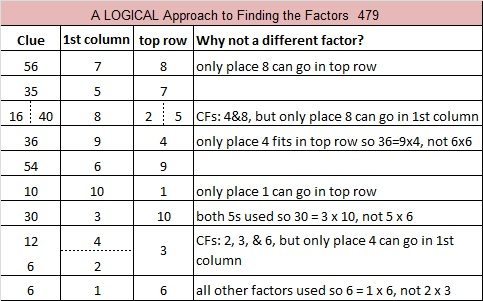# Just a Little 473 Cake

Today is our wedding anniversary. Today I tried to spend my time with my husband instead of the computer. Hence this is a very short and plain post.

The middle digit of 473 equals the sum of the other two digits which means that 473 can be evenly divided by 11.

Five years from now I could make one of these cakes for our anniversary!473 is the sum of some consecutive prime numbers two different ways. See if you can find them yourself. Then check the comments to see if you were right.

• 473 is a composite number.
• Prime factorization: 473 = 11 x 43
• The exponents in the prime factorization are 1 and 1. Adding one to each and multiplying we get (1 + 1)(1 + 1) = 2 x 2 = 4. Therefore 473 has exactly 4 factors.
• Factors of 473: 1, 11, 43, 473
• Factor pairs: 473 = 1 x 473 or 11 x 43
• 473 has no square factors that allow its square root to be simplified. √473 ≈ 21.74856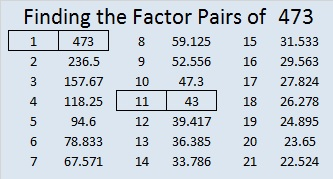# 457 A Pythagorean Triple Logic Puzzle

457 = 4² + 21², and it is the hypotenuse of the primitive Pythagorean triple 168-425-457. Also, 457 is the sum of some consecutive prime numbers. One of my readers posted those primes in the comments.

A long time ago I decided that Pythagorean triples could make a great logic puzzle, so I created one. You can see it directly underneath the following directions:

This puzzle is NOT drawn to scale. Angles that are marked as right angles are 90 degrees, but any angle that looks like a 45 degree angle, isn’t 45 degrees. Lines that look parallel are NOT parallel. Shorter looking line segments may actually be longer than longer looking line segments. Most rules of geometry do not apply here: in fact non-adjacent triangles in the drawing might actually overlap.

No geometry is needed to solve this puzzle. All that is needed is the table of Pythagorean triples under the puzzle. The puzzle only uses triples in which each leg and each hypotenuse is less than 100 units long. The puzzle has only one solution.

If any of these directions are not clear, let me know in the comments. I will NOT be publishing the solution to this puzzle, but I will allow anyone who desires to put any or all of the missing values in the comments. Also, the comments will help me determine if I should publish another puzzle like this one.

Good Luck!Print the puzzles or type the solution on this excel file:  10 Factors 2015-04-13

—————————————————————————————————

• 457 is a prime number.
• Prime factorization: 457 is prime.
• The exponent of prime number 457 is 1. Adding 1 to that exponent we get (1 + 1) = 2. Therefore 457 has exactly 2 factors.
• Factors of 457: 1, 457
• Factor pairs: 457 = 1 x 457
• 457 has no square factors that allow its square root to be simplified. √457 ≈ 21.3776How do we know that 457 is a prime number? If 457 were not a prime number, then it would be divisible by at least one prime number less than or equal to √457 ≈ 21.3776. Since 457 cannot be divided evenly by 2, 3, 5, 7, 11, 13, 17, or 19, we know that 457 is a prime number.

# 449 and Level 1

449 is the sum of consecutive prime numbers. How many and what are they? Check the comments to see the answer.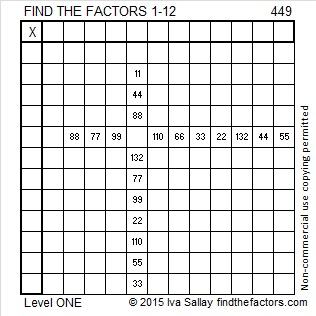Print the puzzles or type the factors on this excel file:  12 Factors 2015-04-06

449 = 20² + 7² so 449 is the hypotenuse of a Pythagorean triple. That triple will be 2(20)(7), 20² – 7², 20² + 7² which is primitive triple 280-351-449.

——————————————————————————————————————-

• 449 is a prime number.
• Prime factorization: 449 is prime.
• The exponent of prime number 449 is 1. Adding 1 to that exponent we get (1 + 1) = 2. Therefore 449 has exactly 2 factors.
• Factors of 449: 1, 449
• Factor pairs: 449 = 1 x 449
• 449 has no square factors that allow its square root to be simplified. √449 ≈ 21.1896How do we know that 449 is a prime number? If 449 were not a prime number, then it would be divisible by at least one prime number less than or equal to √449 ≈ 21.1896. Since 449 cannot be divided evenly by 2, 3, 5, 7, 11, 13, 17, or 19, we know that 449 is a prime number.

——————————————————————————————————————-# 440 Can You Appreciate a Good Paradox?

Brainden.com gives several examples of paradoxes as well as this definition: “A paradox is a statement that contradicts itself or a situation which seems to defy logic.”

Alan Parr suggested I write no more than the fact that 440 is the sum of consecutive prime numbers. It may seem to defy logic that he would want to try to figure out what those consecutive primes are without any hints, but he proved yesterday he is up for the challenge. Check the comments to see if he (or somebody else) can figure out what those prime numbers might be. I am waiting to see if he can again defy logic and figure them out. In the meantime, the factors of 440 will be listed at the end of this post.

I remember watching the episode of Star Trek in which several androids malfunctioned because they couldn’t handle paradoxes, but most humans seem to be able to handle paradoxes just fine. I’ve written one myself that I hope you will enjoy:

I overheard a pair of docs in the middle of a heated argument. One of them said, “As I have stated hundreds of times before, no matter what I say, YOU ALWAYS contradict me!” The other doc shook his head saying, “No, no. That’s not correct at all. YOU’RE the one who’s always contradicting ME!” I hate to hear arguing, so I left, but I have to wonder if they ever worked it out or are they still arguing about contradicting each other even today?

You might not expect to find paradoxes in the The New Testament, but crosswalkblogdotcom describes several of them beautifully in I-will-take-paradox-for-200-alec.

Shakespeare and Wordsworth used them as well. See examples in Is-this-phrase-grammatically-and-semantically-correct?

When I first joined twitter, this is part of the first conversation I had:

Steve Morris has written about paradoxes on several occasions including his very popular post titled Failure-to-fail. If we fail to fail, we become the biggest failure.

It is a paradox to have to be willing to fail in order to really succeed. As Michael Jordan stated in a Nike commercial: “I’ve missed more than 9000 shots in my career. I’ve lost almost 300 games. 26 times, I’ve been trusted to take the game winning shot and missed. I’ve failed over and over and over again in my life. And that is why I succeed.”

We will face setbacks, trials of faith, and other paradoxes in our lives, but we must not give up. All of them are opportunities for us to learn and grow. We actually ought to be grateful for them, because as we face them, we become stronger and more successful. Zeno’s paradox would have us believe that we can never reach our goals. That is simply not true. We can reach them if we keep them in our sights and keep going toward them relentlessly.

Here are the factors of 440:

• 440 is a composite number.
• Prime factorization: 440 = 2 x 2 x 2 x 5 x 11, which can be written 440 = (2^3) x 5 x 11
• The exponents in the prime factorization are 3, 1, and 1. Adding one to each and multiplying we get (3 + 1)(1 + 1)(1 + 1) = 4 x 2 x 2 = 16. Therefore 440 has exactly 16 factors.
• Factors of 440: 1, 2, 4, 5, 8, 10, 11, 20, 22, 40, 44, 55, 88, 110, 220, 440
• Factor pairs: 440 = 1 x 440, 2 x 220, 4 x 110, 5 x 88, 8 x 55, 10 x 44, 11 x 40, or 20 x 22
• Taking the factor pair with the largest square number factor, we get √440 = (√4)(√110) = 2√110 ≈ 20.9762Since 440 = 20 × 22, we know that 441 = 21 × 21.

# 439 and Level 6

439 is a prime number, and it is also the sum of consecutive prime numbers in TWO different ways. Can you find either or both of those ways? You can write your answer or give or ask for hints in the comments.

In case someone is not sure what consecutive prime numbers are, here is an example: 17, 19, 23, 29, 31, and 37 are six consecutive prime numbers because they are ALL the prime numbers from 17 to 37 and they are listed in order. If we took their sum, we would get 156.Print the puzzles or type the factors on this excel file: 12 Factors 2015-03-23

• 439 is a prime number.
• Prime factorization: 439 is prime.
• The exponent of prime number 439 is 1. Adding 1 to that exponent we get (1 + 1) = 2. Therefore 439 has exactly 2 factors.
• Factors of 439: 1, 439
• Factor pairs: 439 = 1 x 439
• 439 has no square factors that allow its square root to be simplified. √439 ≈ 20.9523How do we know that 439 is a prime number? If 439 were not a prime number, then it would be divisible by at least one prime number less than or equal to √439 ≈ 20.9523. Since 439 cannot be divided evenly by 2, 3, 5, 7, 11, 13, 17, or 19, we know that 439 is a prime number.

# 410 Is The Sum of Two Squares Two Different Ways

410 = 59 + 61 + 67 + 71 + 73 + 79, six consecutive prime numbers.

410 = 199 + 211, two consecutive prime numbers.

• 410 is a composite number.
• Prime factorization: 410 = 2 x 5 x 41
• The exponents in the prime factorization are 1, 1, and 1. Adding one to each and multiplying we get (1 + 1)(1 + 1)(1 + 1) = 2 x 2 x 2 = 8. Therefore 410 has exactly 8 factors.
• Factors of 410: 1, 2, 5, 10, 41, 82, 205, 410
• Factor pairs: 410 = 1 x 410, 2 x 205, 5 x 82, or 10 x 41
• 410 has no square factors that allow its square root to be simplified. √410 ≈ 20.2485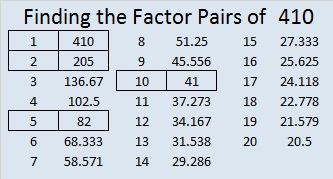Those factors hardly seem like anything to write home about, but wait….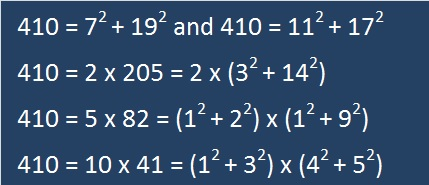How do I know all this? I applied something I read on Dr. David Mitchell’s blog, Lattice Labyrinths. He recently wrote a fascinating post about Tessellations and Pythagorean Triples. You have to read the article or at least look at his tessellations!

I didn’t know multiplying tessellating lattice labyrinths had anything to do with Pythagorean triples, but they do! (Actually I didn’t know anything about tessellating lattice labyrinths until I read the post.)

About the fourth or fifth paragraph of Dr. Mitchell’s post, he states something I didn’t know before: If you take one number that is the sum of two squares and find another number that is also the sum of two squares, and then if you multiply those two numbers together, that product will also be the sums of two squares! Since I knew both 10 and 41 could be written as the sum of two squares, I had to see if 410 could be also. I was doubly surprised and certainly not the least bit disappointed. I don’t know if it’s unusual that most of 410’s factors can also be written as the sum of two squares.

Because 410 and so many of its factors have that property, it is the hypotenuse of four non-primitive Pythagorean triples:

• [266-312-410] is [133 – 156 – 205] times 2.
• [246-328-410] is [3-4-5] times 82.
• [168-374-410] is [84-187-205] times 2.
• [90-400-410] is [9-40-41] times 10.

It is also the short leg of four other triples:

• [410-984-1066] is [5-12-13] times 82.
• [410-1656-1706] is [205-828-853] times 2.
• [410-8400-8410] is [41-840-841] times 10.
• [410-42024-42026] is [205-21012-21013] times 2.